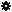﻿ Object Model: NurbsCurve.GetPercentageFromU

NurbsCurve.GetPercentageFromU

## NurbsCurve.GetPercentageFromU### Description

Returns the percentage (with values between 0.0 and 100.0 inclusive) along the curve at the given U value.

### C# Syntax

 ```Double NurbsCurve.GetPercentageFromU( Double in_dUValue ); ```

### Scripting Syntax

 ```oDouble = NurbsCurve.GetPercentageFromU( UValue ); ```

### Parameters

Parameter Type Description
UValue Double The U value at which we want to evaluate the curve.

### Examples

#### VBScript Example

 ```set oRoot = Application.ActiveProject.ActiveScene.Root set oArc = oRoot.AddGeometry( "Arc", "NurbsCurve" ) percentage = oArc.ActivePrimitive.Geometry.Curves(0).GetPercentageFromU( 2.0 ) LogMessage "The percenatage of the curve at U = 2.0 is :" & percentage ```